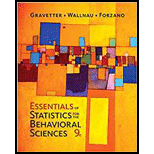Chapter 14, Problem 22PEssentials of Statistics for The B...

9th Edition
Frederick J Gravetter + 2 others
ISBN: 9781337098120

Solutions

Chapter
SectionEssentials of Statistics for The B...

9th Edition
Frederick J Gravetter + 2 others
ISBN: 9781337098120
Textbook Problem

The regression equation is computed for a set of n = 18 pairs of X and Y values with a correlation of r = +0.50 and SSY = 48. a. Find the standard error of estimate for the regression equation. b. How big would the standard error be if the sample size were n- 66?

a.

To determine

To find: The standard error of estimate for the regression equation.

Explanation

Given info:

The regression equation is computed for a set of n=18 pairs of X and Y values with a correlation of r=0.50 and SSy=48 .

Calculation:

The standard error of estimate is calculated as:

SE=SSresidualdf

Here SE is for standard error of estimate and df is degree of freedom calculated as:

df=n2=182=16

For calculating the standard error of estimates for the regression equation need to calculate SSresidual.. The SS residual also known as unpredicted variability is calculated as:

SSresidual=(1r2)SSy

Where r is given as 0.50, so r2 is calculated as 0

a.

To determine
The standard error if the sample size is n=66 .

Still sussing out bartleby?

Check out a sample textbook solution.

See a sample solution

The Solution to Your Study Problems

Bartleby provides explanations to thousands of textbook problems written by our experts, many with advanced degrees!

Get Started

Expand each expression in Exercises 122. (y1y)(y+1y)

Finite Mathematics and Applied Calculus (MindTap Course List)

Divide: 84

Elementary Technical Mathematics

True or False: The function graphed at the right is one-to-one.

Study Guide for Stewart's Single Variable Calculus: Early Transcendentals, 8th

Given: Line l and point P not on l Construct: PQl

Elementary Geometry for College Students